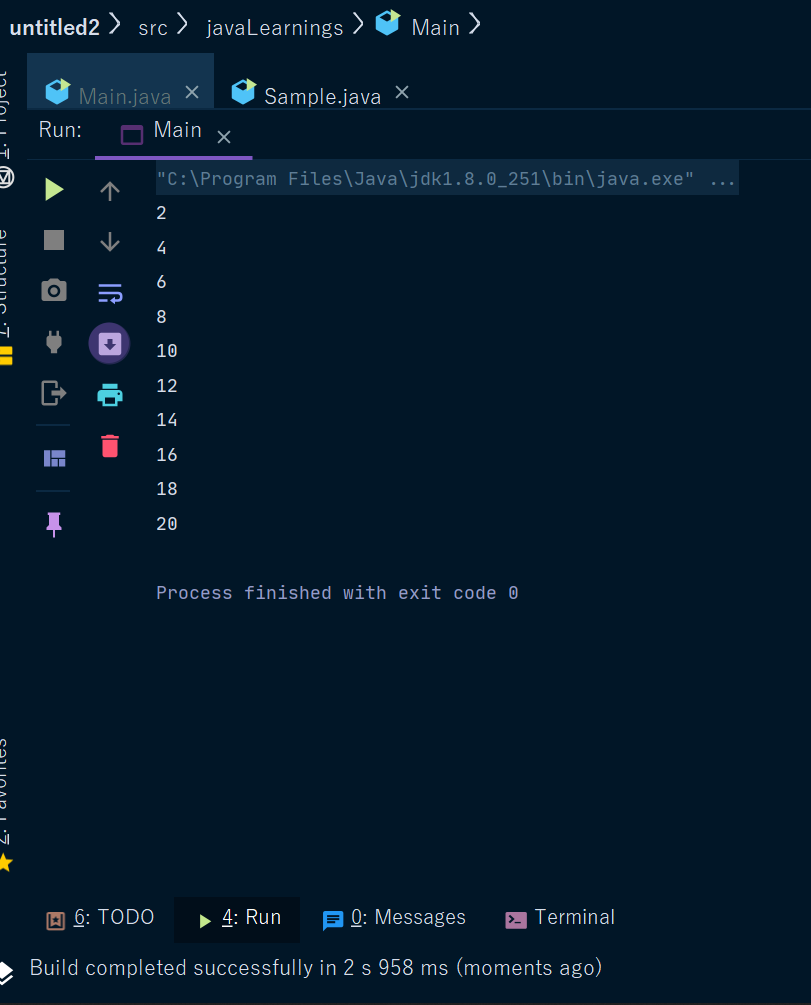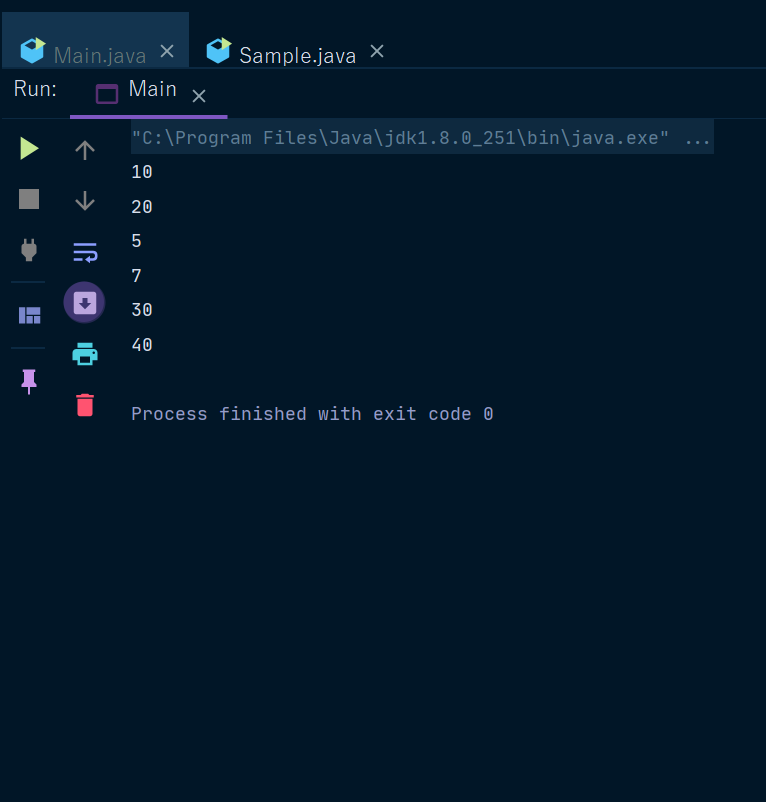Java For Keyword

# Java For Keyword

For Keyword In Java
For keyword is used to define for-loops in Java, and basically in all programming languages.
It is used for fixed iterations.
For loop is the most commonly used form of iteration.
This loop performs initialization before the first iteration. Then it performs conditional testing and, at the end of each iteration, some stepping happens.
Now we will see examples -
Example1- Write a program that writes your name 100 times on screen.
 class T { public static void main(string[] args) { int i; for(i=1;i<=100;i++){ system.out.print("NAME"); } }
Example2 - Write a program that displays a table of 2.
 class T { public static void main(string[] args) { int i; for(i=2;i<=20;i=i+2){ System.out.println(i); } } —------------------------------------------ Another way - class T { public static void main(string[] args) { int i,a=2; for(i=1;i<=10;i++){ System.out.println(i*2); } }
Output -New Variation of for- loop in Java-
• Also known as for-each-loop.
• It is used to traverse an array.
Syntax -
 for(datatype variableName : ArrayName) {     statement; }
Now we will see an example -
Example -
 int a[] = {10,20,5,7,30,40}; for(int b : a) System.out.println(b);
Output -